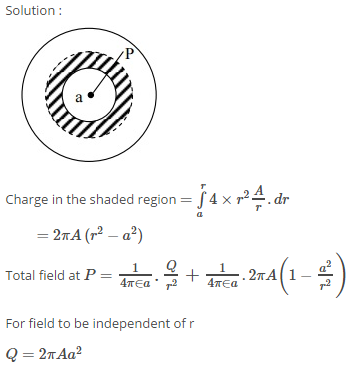# the region between two concentric spheres of radii 'a' and 'b' respectively , has a volume charge density rho=A/r , where r is the distance from the centre and A is constant . at the centre of the spheres is a point charge Q. The value of A such that the electric field in the region between the spheres will be constant is

Dear Student,

According to Gauss's Law, if we consider the gaussian surface with radius a, then the electric field at that point is,

$E.\left(4\pi {a}^{2}\right)=\frac{Q}{{\epsilon }_{0}}⇒E=\frac{Q}{4\pi {\epsilon }_{0}{a}^{2}}$

Now, if we consider the gaussian surface with the radius b, then the electric field at that point is,

$E.\left(4\pi {b}^{2}\right)=\frac{Q+{\int }_{a}^{b}\frac{A}{r}4\pi {r}^{2}dr}{{\epsilon }_{0}}⇒E=\frac{Q+4\pi A{\int }_{a}^{b}rdr}{4\pi {\epsilon }_{0}{b}^{2}}\phantom{\rule{0ex}{0ex}}⇒E=\frac{Q+2\pi A\left({b}^{2}-{a}^{2}\right)}{4\pi {\epsilon }_{0}{b}^{2}}$

Given that the electric field should be constant. Therefore,

• 1A = Q / 2 pi a2
• 2
What are you looking for?